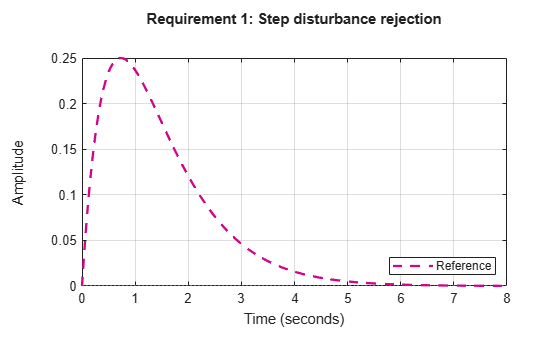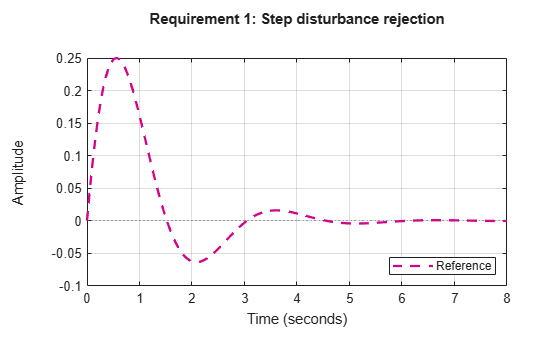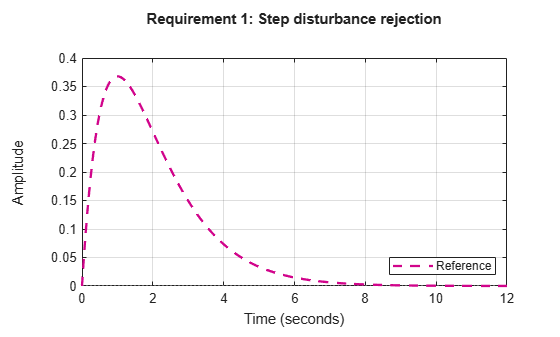# TuningGoal.StepRejection

Step disturbance rejection requirement for control system tuning

## Description

Use `TuningGoal.StepRejection` to specify how a step disturbance injected at a specified location in your control system affects the signal at a specified output location. Use this tuning goal with control system tuning commands such as `systune` or `looptune`.

You can specify the desired response in time-domain terms of peak value, settling time, and damping ratio. Alternatively, you can specify the response as a stable reference model having DC-gain. In that case, the tuning goal is to reject the disturbance as well as or better than the reference model.

To specify disturbance rejection in terms of a frequency-domain attenuation profile, use `TuningGoal.Rejection`.

## Creation

### Syntax

``Req = TuningGoal.StepRejection(inputname,outputname,refsys)``
``Req = TuningGoal.StepRejection(inputname,outputname,peak,tSettle)``
``Req = TuningGoal.StepRejection(inputname,outputname,peak,tSettle,zeta)``

### Description

example

````Req = TuningGoal.StepRejection(inputname,outputname,refsys)` creates a tuning goal that constrains how a step disturbance injected at a location `inputname` affects the response at `outputname`. The tuning goal is that the disturbance be rejected as well as or better than the reference system. `inputname` and `outputname` can describe a SISO or MIMO response of your control system. For MIMO responses, the number of inputs must equal the number of outputs.```
````Req = TuningGoal.StepRejection(inputname,outputname,peak,tSettle)` specifies an oscillation-free response in terms of a peak value and a settling time. ```
````Req = TuningGoal.StepRejection(inputname,outputname,peak,tSettle,zeta)` allows for damped oscillations with a damping ratio of at least `zeta`. ```

### Input Arguments

expand all

Input signals for the tuning goal, specified as a character vector or, for multiple-input tuning goals, a cell array of character vectors.

• If you are using the tuning goal to tune a Simulink® model of a control system, then `inputname` can include:

• Any model input.

• Any linear analysis point marked in the model.

• Any linear analysis point in an `slTuner` (Simulink Control Design) interface associated with the Simulink model. Use `addPoint` (Simulink Control Design) to add analysis points to the `slTuner` interface. Use `getPoints` (Simulink Control Design) to get the list of analysis points available in an `slTuner` interface to your model.

For example, suppose that the `slTuner` interface contains analysis points `u1` and `u2`. Use `'u1'` to designate that point as an input signal when creating tuning goals. Use `{'u1','u2'}` to designate a two-channel input.

• If you are using the tuning goal to tune a generalized state-space (`genss`) model of a control system, then `inputname` can include:

For example, if you are tuning a control system model, `T`, then `inputname` can be any input name in `T.InputName`. Also, if `T` contains an `AnalysisPoint` block with a location named `AP_u`, then `inputname` can include `'AP_u'`. Use `getPoints` to get a list of analysis points available in a `genss` model.

If `inputname` is an `AnalysisPoint` location of a generalized model, the input signal for the tuning goal is the implied input associated with the `AnalysisPoint` block:For more information about analysis points in control system models, see Mark Signals of Interest for Control System Analysis and Design.

Output signals for the tuning goal, specified as a character vector or, for multiple-output tuning goals, a cell array of character vectors.

• If you are using the tuning goal to tune a Simulink model of a control system, then `outputname` can include:

• Any model output.

• Any linear analysis point marked in the model.

• Any linear analysis point in an `slTuner` (Simulink Control Design) interface associated with the Simulink model. Use `addPoint` (Simulink Control Design) to add analysis points to the `slTuner` interface. Use `getPoints` (Simulink Control Design) to get the list of analysis points available in an `slTuner` interface to your model.

For example, suppose that the `slTuner` interface contains analysis points `y1` and `y2`. Use `'y1'` to designate that point as an output signal when creating tuning goals. Use `{'y1','y2'}` to designate a two-channel output.

• If you are using the tuning goal to tune a generalized state-space (`genss`) model of a control system, then `outputname` can include:

For example, if you are tuning a control system model, `T`, then `outputname` can be any output name in `T.OutputName`. Also, if `T` contains an `AnalysisPoint` block with a location named `AP_u`, then `outputname` can include `'AP_u'`. Use `getPoints` to get a list of analysis points available in a `genss` model.

If `outputname` is an `AnalysisPoint` location of a generalized model, the output signal for the tuning goal is the implied output associated with the `AnalysisPoint` block:For more information about analysis points in control system models, see Mark Signals of Interest for Control System Analysis and Design.

Reference system for target step rejection, specified as a SISO dynamic system model, such as a `tf`, `zpk`, or `ss` model. `refsys` must be stable and proper, and must have zero DC gain. This restriction ensures perfect rejection of the steady-state disturbance.

`refsys` can be continuous or discrete. If `refsys` is discrete, it can include time delays which are treated as poles at `z` = 0.

For best results, `refsys` and the open-loop response from the disturbance to the output should have similar gains at the frequency where the reference model gain peaks. You can check the peak gain and peak frequency using `getPeakGain`. For example:

`[gmax,fmax] = getPeakGain(refsys);`

Use `getIOTransfer` to extract the corresponding open-loop response from the system you are tuning.

Peak absolute value of target response to disturbance, specified as a scalar value.

Target settling time of the response to disturbance, specified as a positive scalar value, in the time units of the control system you are tuning.

Minimum damping ratio of oscillations in the response to disturbance, specified as a value between 0 and 1.

## Properties

expand all

Reference system for target response to step disturbance, specified as a SISO (`zpk`) model. The step response of this model specifies how the output signals specified by `outputname` should respond to the step disturbance at `inputname`.

If you use the `refsys` input argument to create the tuning goal, then the value of `ReferenceModel` is `zpk(refsys)`.

If you use the `peak`, `tSample`, and `zeta` input arguments, then `ReferenceModel` is a `zpk` representation of the first-order or second-order transfer function whose step response has the specified characteristics.

Input signal scaling, specified as a vector of positive real elements.

Use this property to specify the relative amplitude of each entry in vector-valued input signals when the choice of units results in a mix of small and large signals. This information is used to scale the closed-loop transfer function from `Input` to `Output` when the tuning goal is evaluated.

Suppose T(s) is the closed-loop transfer function from `Input` to `Output`. The tuning goal is evaluated for the scaled transfer function Do–1T(s)Di. The diagonal matrices Do and Di have the `OutputScaling` and `InputScaling` values on the diagonal, respectively.

The default value, `[]` , means no scaling.

Output signal scaling, specified as a vector of positive real values.

Use this property to specify the relative amplitude of each entry in vector-valued output signals when the choice of units results in a mix of small and large signals. This information is used to scale the closed-loop transfer function from `Input` to `Output` when the tuning goal is evaluated.

Suppose T(s) is the closed-loop transfer function from `Input` to `Output`. The tuning goal is evaluated for the scaled transfer function Do–1T(s)Di. The diagonal matrices Do and Di have the `OutputScaling` and `InputScaling` values on the diagonal, respectively.

The default value, `[]` , means no scaling.

Names of disturbance input locations, specified as a cell array of character vectors. This property is initially populated by the `inputname` argument when you create the tuning goal.

Names of locations at which response to step disturbance is measured, specified as a cell array of character vectors. This property is initially populated by the `outputname` argument when you create the tuning goal.

Models to which the tuning goal applies, specified as a vector of indices.

Use the `Models` property when tuning an array of control system models with `systune`, to enforce a tuning goal for a subset of models in the array. For example, suppose you want to apply the tuning goal, `Req`, to the second, third, and fourth models in a model array passed to `systune`. To restrict enforcement of the tuning goal, use the following command:

`Req.Models = 2:4;`

When `Models = NaN`, the tuning goal applies to all models.

Feedback loops to open when evaluating the tuning goal, specified as a cell array of character vectors that identify loop-opening locations. The tuning goal is evaluated against the open-loop configuration created by opening feedback loops at the locations you identify.

If you are using the tuning goal to tune a Simulink model of a control system, then `Openings` can include any linear analysis point marked in the model, or any linear analysis point in an `slTuner` (Simulink Control Design) interface associated with the Simulink model. Use `addPoint` (Simulink Control Design) to add analysis points and loop openings to the `slTuner` interface. Use `getPoints` (Simulink Control Design) to get the list of analysis points available in an `slTuner` interface to your model.

If you are using the tuning goal to tune a generalized state-space (`genss`) model of a control system, then `Openings` can include any `AnalysisPoint` location in the control system model. Use `getPoints` to get the list of analysis points available in the `genss` model.

For example, if `Openings = {'u1','u2'}`, then the tuning goal is evaluated with loops open at analysis points `u1` and `u2`.

Name of the tuning goal, specified as a character vector.

For example, if `Req` is a tuning goal:

`Req.Name = 'LoopReq';`

## Examples

collapse all

Create a requirement that specifies the step disturbance response in terms of peak time-domain response, settling time, and damping of oscillations.

Suppose you want the response at `'y'` to a disturbance injected at `'d'` to never exceed an absolute value of 0.25, and to settle within 5 seconds. Create a `TuningGoal.StepRejection` requirement that captures these specifications and also specifies non-oscillatory response.

`Req1 = TuningGoal.StepRejection('d','y',0.25,5);`

Omitting an explicit value for the damping ratio, `zeta`, is equivalent to setting `zeta` = 1. Therefore, `Req` specifies a non-oscillatory response. The software converts the peak value and settling time into a reference transfer function whose step response has the desired time-domain profile. This transfer function is stored in the `ReferenceModel` property of `Req`.

`Req1.ReferenceModel`
```ans = 0.92883 s ----------- (s+1.367)^2 Continuous-time zero/pole/gain model. ```

Confirm the target response by displaying `Req`.

```figure() viewGoal(Req1)```Suppose your application can tolerate oscillations provided the damping ratio is less than 0.4. Create a requirement that specifies this disturbance response.

```Req2 = TuningGoal.StepRejection('d','y',0.25,5,0.4); figure() viewGoal(Req2)```Create a requirement that specifies the step disturbance response as a transfer function.

Suppose you want the response to a disturbance injected at an analysis point `d` in your control system and measured at a point `'y'` to be rejected at least as well as the transfer function

`$H\left(s\right)=\frac{s}{{s}^{2}+2s+1}.$`

Create a `TuningGoal.StepRejection` requirement.

```H = tf([1 0],[1 2 1]); Req = TuningGoal.StepRejection('d','y',H);```

Display the requirement.

`viewGoal(Req)`The plot displayed by `viewGoal` shows the step response of the specified transfer function. This response is the target time-domain response to disturbance.

## Tips

• This tuning goal imposes an implicit stability constraint on the closed-loop transfer function from `Input` to `Output`, evaluated with loops opened at the points identified in `Openings`. The dynamics affected by this implicit constraint are the stabilized dynamics for this tuning goal. The `MinDecay` and `MaxRadius` options of `systuneOptions` control the bounds on these implicitly constrained dynamics. If the optimization fails to meet the default bounds, or if the default bounds conflict with other requirements, use `systuneOptions` to change these defaults.

## Algorithms

When you tune a control system using a `TuningGoal`, the software converts the tuning goal into a normalized scalar value f(x), where x is the vector of free (tunable) parameters in the control system. The software then adjusts the parameter values to minimize f(x) or to drive f(x) below 1 if the tuning goal is a hard constraint.

`TuningGoal.StepRejection` aims to keep the gain from disturbance to output below the gain of the reference model. The scalar value of the tuning goal f(x) is given by:

`$f\left(x\right)={‖{W}_{F}\left(s\right){T}_{dy}\left(s,x\right)‖}_{\infty },$`

or its discrete-time equivalent. Here, Tdy(s,x) is the closed-loop transfer function from `Input` to `Output`, and ${‖\text{\hspace{0.17em}}\cdot \text{\hspace{0.17em}}‖}_{\infty }$ denotes the H norm (see `norm`). WF is a frequency weighting function derived from the step-rejection profile you specify in the tuning goal. The gains of WF and `1/ReferenceModel` roughly match for gain values within 60 dB of the peak gain. For numerical reasons, the weighting function levels off outside this range, unless you specify a reference model that changes slope outside this range. This adjustment is called regularization. Because poles of WF close to s = 0 or s = `Inf` might lead to poor numeric conditioning of the `systune` optimization problem, it is not recommended to specify reference models with very low-frequency or very high-frequency dynamics.

To obtain WF, use:

`WF = getWeight(Req,Ts)`

where `Req` is the tuning goal, and `Ts` is the sample time at which you are tuning (`Ts = 0` for continuous time). For more information about regularization and its effects, see Visualize Tuning Goals.

## Version History

Introduced in R2016a

expand all